## Main

Although density functional theory has been the workhorse of computational materials science for several decades1, systematic routes to improve the crucial but approximate exchange-correlation functionals do not exist2. In contrast, for molecular systems, a systematic hierarchy of approximate yet highly successful quantum-chemical techniques, such as coupled-cluster theory, has long been established3. This hierarchy has not yet been explored in solids, although initial implementations of its lower levels have been encouraging4,5,6,7,8,9,10,11. Part of the reason for this is the high computational complexity of quantum-chemical methods. The computational cost grows rapidly with the number of considered electrons N and with the basis set size M. Traditional full configuration interaction (FCI), that is, exact diagonalization, has combinatorial scaling and can cope with at most some ten electrons in a small basis; and even coupled-cluster methods, although requiring only a computational time that is polynomial in M and N, are extremely expensive. However, recent developments in methodology, as well as the increase in computer power, mean that it is now possible to address their accuracy and applicability in this domain.

In the development of quantum chemistry, FCI has played an invaluable benchmarking role, by providing exact results within a given basis. This has enabled the electron correlation problem to be addressed in isolation from other complicating factors inherent when comparing to experiment12. Moreover, FCI enables us to assess the degree to which the electronic wavefunction is dominated by a single determinant, and therefore which systems are likely to be well described by approximations such as many-body perturbation theory and coupled-cluster theory13. In solids, however, the absence of FCI means that the accuracy of such approximations cannot be easily gauged, especially in systems where correlations are expected to be strong. Here we provide, for the first time to our knowledge, FCI-quality energies in a range of realistic solids and unambiguously evaluate the accuracy of high-level quantum-chemical methods.

A further motivation for the application of quantum-chemical methods to solids comes from the multitude of recent developments, which hold the promise to reduce the computational cost beyond our present consideration. These include optimized virtual spaces14, explicit correlation15,16, exploitation of locality of correlation5, and others17,18,19, and should be directly transferable to the solid state. Combining high accuracy with increased efficiency, quantum-chemistry methods hold the promise to routinely bring high accuracy to computational materials science.

## FCIQMC and the quantum-chemical hierarchy

The FCI quantum Monte Carlo (FCIQMC) method has emerged as a tool to calculate energies that are essentially identical to the true correlation energy captured by the basis set, whilst having a significantly lower computational scaling than a traditional brute force diagonalization of the problem (FCI). This makes it ideally suited for a systematic benchmarking of wavefunction-based methods in the solid state20,21,22,23,24,25. This method involves a stochastic sampling of a Slater determinant space constructed from the basis set—a function space of orthonormal antisymmetrized determinants in which the wavefunctions are expressed. This method has previously been applied to molecular systems20,21,22,23,24 and the homogeneous electron gas25, where energies were calculated that compared favourably, or in some cases surpassed in accuracy, those achieved with state-of-the-art diffusion Monte Carlo (DMC) techniques26. This provides the confidence to tackle more realistic solid-state systems here.

Although it is possible for DMC to be used as a benchmark for quantum-chemistry methods and vice versa27, DMC does not operate in a Slater determinant space, but rather a real space representation of the wavefunction. As such, it would require quantum-chemical calculations to be converged to high accuracy with respect to the basis set size before any meaningful comparisons could be drawn. Instead, by comparing to values obtained in the same Slater determinant basis, robust comparisons between FCIQMC and more approximate diagrammatic methods can be drawn without the need for absolute convergence. More approximate methods can then also be used to extrapolate to the infinite basis set limit14,17,28. Additionally, DMC requires an approximation for the nodal surface of the wavefunction. Although this error can be made relatively small29,30, releasing the nodal surface is notoriously difficult for solids and greatly increases the computational demand. Similarly, another variant of QMC methods, auxiliary-field quantum Monte Carlo (AFQMC), although now operating in a space of Slater determinants and with favourable scaling, requires analogous constraints within the phaseless approximation in order to go to realistic system sizes and avoid transient energy estimates31,32.

Here we illustrate the extension of the FCIQMC method to the solid state. The introduction of translational and crystal momentum symmetries, which arise from working with finite simulation cells with periodic boundary conditions, necessitates a change to the ‘walker’ dynamics and ‘initiator’ rules (see below for descriptions). In order to take advantage of these properties, the method is reformulated for complex wavefunctions. The presence of a ‘phase’ problem, rather than a simpler sign problem, is considered. A range of systems, from the extensively studied lithium hydride, to other ionic, covalent and rare gas solids is considered, while benchmarking the established quantum-chemical methods of second-order Møller–Plesset theory (MP2)33, coupled-cluster singles and doubles (CCSD)34, and the first implementation of perturbative triples (CCSD(T))35 for periodic systems. These three methods are generally considered to possess a favourable trade-off between accuracy and cost, and are the most widely used of all quantum-chemical methods. Finally, we study the far more complex electronic structure of the charge-transfer solid NiO, to evaluate the ability of these quantum-chemical methods to handle strong correlation effects, where deficiencies are likely to be exposed.

## Sampling in the solid state

The recently developed FCIQMC method20,21,22,23,24 involves a discrete sampling of the wavefunction by signed ‘walkers’ which stochastically evolve within a Hilbert space of N-electron Slater determinants as illustrated in Fig. 1. The method converges rapidly with the number of walkers to the FCI limit, and generally the number of walkers required is a tiny fraction of the Hilbert space. This leads to a huge compression in the wavefunction information, while correctly reproducing exact time-averaged properties. The reason that a sufficient number of walkers is required has to do with overcoming the ‘fermion sign problem’ present in the Monte Carlo sampling of any fermionic wavefunction36. The sign problem in this space manifests itself in the presence of a lower-energy state characterized by a combination of the ±Ψ degenerate solutions, which if not suppressed, leads to an exponential increase in noise. The growth of this state is controlled in FCIQMC by annihilation between walkers of opposite signs, which stabilizes the wavefunction to one signed solution20,37. The discrete space of Slater determinants allows FCIQMC to implement this annihilation exactly, and provided the walker population is large enough, will directly overcome the sign problem without requiring constraints on the wavefunction.

Because walker annihilation can only occur on occupied determinants, it is important to ensure that the newly occupied space remains sign-coherent to the currently sampled wavefunction. This is the rationale behind the ‘initiator’ rules used in the i-FCIQMC method22, which is used exclusively in this work, whereby newly occupied determinants must have originated from a determinant with a population greater than a parameter nadd. By restricting the growth of the occupied space in this way, the walker density and hence annihilation rate is kept high, ensuring that propagation of noise in the system is kept to a minimum. This biases the dynamic in a small way, but rigorously converges onto exact energies of the Hamiltonian as the walker number increases.

The determinants in this work are composed from antisymmetrized products of one-electron orbitals obtained from a prior Hartree–Fock calculation in a large basis of periodic plane waves within the framework of the projector-augmented wave method, as implemented in VASP. If these orbitals are strictly real then the wavefunction Ψ can also be real20. In this work, however, the orbitals are complex Bloch functions, to account for the translational invariance of the potential. With these we can construct many body wavefunctions and use k-point sampling to ensure convergence, rather than sampling ever larger unit cells to remove finite-size effects.

Because it is necessary to correlate between sampled k-points, the number of explicitly correlated electrons and orbitals increases linearly with the number of sampled k-points yielding combinatorial scaling in the size of the Hilbert space (essentially exponential with the number of k-points). However, performing this sampling increases the number of zero Hamiltonian matrix elements between determinants, as crystal momentum must be conserved. By implementing an algorithm to stochastically generate only these momentum-allowed excitations, a saving that grows quadratically with the number of k-points is achieved, because both the accessible space is reduced, and the magnitude of the time step is increased.

To take advantage of these savings, it is necessary to work with complex orbitals, requiring a complementary set of both ‘real’ and ‘imaginary’ walkers in the FCIQMC dynamic, and a reformulation of the algorithm. The master equations of the FCIQMC method follow naturally from the imaginary-time Schrödinger equation, and are given by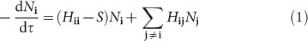where Ni represents the now complex walker population on determinant Di, τ represents imaginary time, S is a strictly real energy-offset parameter denoted the ‘shift’, which controls population growth, and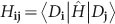is the many-electron Hamiltonian evaluated between two determinants. In each iteration, for each walker (real and imaginary) on a determinant Di, a suitable momentum-allowed excitation, Dj, is generated. The real (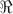) and imaginary (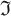) parts of Hij are considered in turn, and two attempts at generating new walkers on Dj are stochastically realized. For real parent walkers: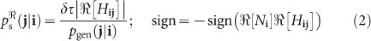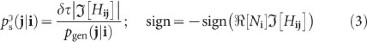and for imaginary parent walkers: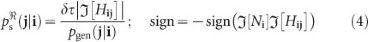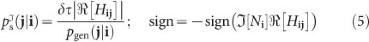where τ is the timestep for the simulation, and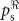and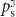indicate the probability of creating real and imaginary child walkers, respectively. pgen(j|i) is the probability of generating determinant Dj from Di. After this step, the ‘death’ step is performed for each occupied determinant, with the same death probability for the real and imaginary walkers of δτ(Hii − E0 − S) stochastically realized, where E0 is a reference energy, and Hii is now strictly real. A final annihilation step occurs every iteration, where real and imaginary walkers are separately considered, and pairs of opposite sign on the same determinant are removed from the simulation.

The value of S can be used as a strictly real measure of the correlation energy of the problem; however, provided a good overlap of the walker distribution with a reference wavefunction D0 (generally taken to be the Hartree–Fock determinant) can be found, an averaged projected estimator is often less noisy: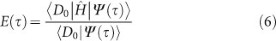where 〈D0|Ψ(τ)〉 = N0. As opposed to S, E(τ) is now a complex quantity, where in order to achieve real energies, the imaginary part of the energy must cancel to zero in a non-trivial way. In order to test this, we considered rock-salt-structured LiH sampled using 2 × 2 × 2 k-points. By choosing all k-points to lie at the Γ-point or Brillouin zone boundary, it was possible to take linear combinations of the orbitals to give a strictly real basis. This is compared to the complex basis in Fig. 2.Figure 2: Comparison of real and complex i-FCIQMC dynamics for the correlation energy of LiH.

It can be seen that all methods, including FCIQMC, agree exactly between the two bases, and that in the complex basis, the imaginary component of the energy converges to zero within small error bars. Although there is the potential for rotations of the wavefunction in the complex plane, it is observed that the discretization of the wavefunction amplitudes prevents this from happening, and global U(1) transformations are thus suppressed after an initial arbitrary phase factor is determined. This indicates that there is no more of a sign-issue to overcome with annihilation events than that of the original real formulation of the dynamics.

## Real solids and the accuracy of quantum chemistry

Having established the accuracy and efficiency of the complex FCIQMC walker dynamics, it was initially tested on the most widely studied solid to date, rock-salt-structured LiH (refs 6–9, 11, 17, 27). We first benchmark the accuracy of the MP2, CCSD and CCSD(T) energies for this system, by considering the deviation of each from FCIQMC values. Figure 3 shows an equation of state for a range of volumes, with a 3 × 3 × 3 k-point sampling, in a minimal basis required to capture any non-dynamic correlation.Figure 3: Equation of state for rock-salt LiH, with the error from the i-FCIQMC value per unit cell at each volume shown below, for MP2, CCSD and CCSD(T).

The efficient sampling of the i-FCIQMC method is clear, where the 3 × 3 × 3 k-point mesh correlates 54 electrons in a space of 1030 determinants, with convergence to the exact energy obtained after only 50 million walkers, as demonstrated in Fig. 4a. The MP2 values are clearly shifted to higher energies compared to FCIQMC, and because this error changes significantly with volume, the MP2 equilibrium volume and bulk modulus deviate by 3.5% and 6.5%, respectively, from the FCIQMC values.Figure 4: Convergence with respect to the total walker number and k -point meshes.

CCSD energies are virtually parallel to the FCIQMC results, yielding a similar volume and bulk modulus as FCIQMC. Finally, CCSD(T) shows almost exact agreement with FCIQMC in the absolute energies. Overall, these results very much mimic the performance of the standard quantum-chemical hierarchy established for molecular systems and are indicative of their suitability for other similar solids.

To cover different bonding situations, in Fig. 5 we consider the relative errors of quantum-chemical methods for the correlation energies of several crystals when compared to FCIQMC. MP2 and CCSD recover between 80% and 98% of the FCIQMC correlation energy for the sample of rare gas, covalent and ionic solids. The dependence of the relative errors on the various systems is most pronounced in the case of MP2 theory. This is not unexpected and reflects the limitations of low-order perturbation theory. MP2 is more accurate for wide-gap insulators, such as Ne and LiF, than for semiconductors with a smaller gap, like Si and AlP. In contrast, CCSD(T) is shown to give a balanced description across the different systems and is in error by at most 2%.Figure 5: Relative errors compared to i-FCIQMC in a range of solids.

What accuracy, therefore, can one expect from converged CCSD(T) calculations for solids? To answer this question, we computed cohesive energies. These are extremely demanding quantities for any theory, because the correlation effects in solids differ markedly from atoms, potentially leading to large errors in the prediction of the cohesive energy. Furthermore, attention needs to be paid to finite-size scaling (that is, k-point sampling). For the present study we have limited our attention to four solids, rock-salt LiH, diamond, zinc-blende BN and AlP, expecting similar results for the other materials. We have used the progressive downsampling technique14,28, employing k-point meshes of up to 4 × 4 × 4. Figure 4b shows for diamond that the MP2 cohesive energy converges as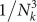, where Nk is the number of k-points used to sample the Brillouin zone in each direction. By fitting to the MP2, CCSD and CCSD(T) energies for 3 × 3 × 3 and 4 × 4 × 4 k-points, we can extrapolate to infinitely dense k-point meshes. The remaining finite size error on the correlation energy is expected to be less than 20 meV per atom for the considered systems. The computational cost is of the order of 25,000 CPU (central processing unit) hours for diamond, with results reported in Table 1.

As anticipated (owing to the established agreement with i-FCIQMC in smaller bases and supercells), the CCSD(T) results are all in almost exact agreement with the experimental cohesive energy corrected for the zero-point energy38. The MP2 cohesive energies generally show substantial error compared to experiment5,9,17. MP2 severely underestimates the correlation energy of atoms, and while it also underestimates the correlation energy of the solids as shown in Fig. 5, the perturbative nature of the theory leads to less underestimation of the correlation energy in solids so that the cohesive energy is often—but not always—overestimated compared to the experimental value. This makes MP2 an unreliable method for solids, especially for calculation of cohesive energies. CCSD and CCSD(T), on the other hand, are far more consistent. Although the absolute errors in the CCSD cohesive energies are still quite sizeable, the cohesive energies are always underestimated. This results from the CCSD correlation energy in the solids being always too small (Fig. 5) whereas in atoms it is generally a good approximation. On adding the perturbative triples correction to the CCSD, the cohesive energies dramatically improve, to an error of only 0.03 eV. As for the absolute correlation energies in solids, the (T) correction over-compensates, leading to too-negative correlation energies, and hence an overestimation of the cohesive energies, albeit only mildly so. We finally note that our results indicate that the residual errors are dominated by correlation errors in the solid, whereas correlation energies for atoms are essentially converged at the CCSD(T) level.

The conclusion is that the accuracy of CCSD(T) has been established for solids to be of the order of 0.03 eV or 1 kcal mol−1. By contrast, the most widely used density functional (PBE39) exhibits a mean-absolute error of 0.15–0.2 eV for a similar range of insulating solids40.

## Towards strong correlation

An important and open question is the domain of applicability of CCSD and CCSD(T) as stronger correlation effects set in. An initial indication of this can be found by comparing the performance of the methods for the spin gap between the ground antiferromagnetic state (AFII) and the ferromagnetic state (FM) of nickel oxide in a rhombohedral unit cell. This is a classic charge-transfer insulator, and it is expected to have strong correlation effects for at least one of the states, and as such, a balanced description for the calculation of the spin gap is expected to provide a stern test.

The results for the FM–AFII spin gap are given in Fig. 6 and illustrate the systematic convergence of the quantum-chemical hierarchy with respect to the correlation treatment of the system. Analysis of the FCIQMC wavefunction indicates that the ground state (AFII) is more strongly correlated and multiconfigurational, with a normalized Hartree–Fock weight of only 0.69, compared to 0.86 for the FM state. This lack of a dominant single reference leads to errors in MP2 of over 50%, while CCSD is still 19% in error compared to i-FCIQMC. Despite this, the qualitative behaviour of the quantum chemical hierarchy remains intact, with CCSD(T) providing an excellent approximation to the exact result. Results from unrestricted Hartree–Fock (UHF) calculations of NiO converged to the thermodynamic limit only capture 17% of the experimental spin gap between the two states41, inferred from neutron scattering42. This agrees well with our restricted k-point sampled system, where the UHF captures 23% of the spin gap compared to FCIQMC results.Figure 6: Energies of the NiO spin gap, calculated at the level of UHF, UMP2, UCCSD, UCCSD(T) and i-FCIQMC in an UHF basis for both spin species.

## Conclusions and outlook

We have shown that FCI-quality correlation energies can be obtained for solid-state systems using an extension of the i-FCIQMC method to complex wavefunctions. We have demonstrated that the standard quantum-chemical hierarchy of increasingly accurate polynomially scaling methods holds for a range of materials, including rare gas, ionic and covalent solids and the charge-transfer insulator NiO. As explicitly shown by the cohesive energies of LiH, C, AlP and BN, CCSD(T) is very accurate for the solid state, surpassing 1 kcal mol−1 accuracy in reproducing experimental results. Considering the proven reliability of CCSD(T) for molecules, we expect a similar precision for insulators and semiconductors in general, with metals possibly requiring further methodological improvements. In combination with recent developments to reduce the computational cost—for instance with explicit inclusion of the cusp condition for the many-electron wavefunction—as well as further technical, algorithmic and methodological advances, the accuracy of FCIQMC and the quantum-chemistry methods will be brought routinely to solid-state physics and computational materials science. We are witnessing a slow but steady change of our computational paradigm.